Ex 11.4

Chapter 11 Class 7 Perimeter and Area
Serial order wise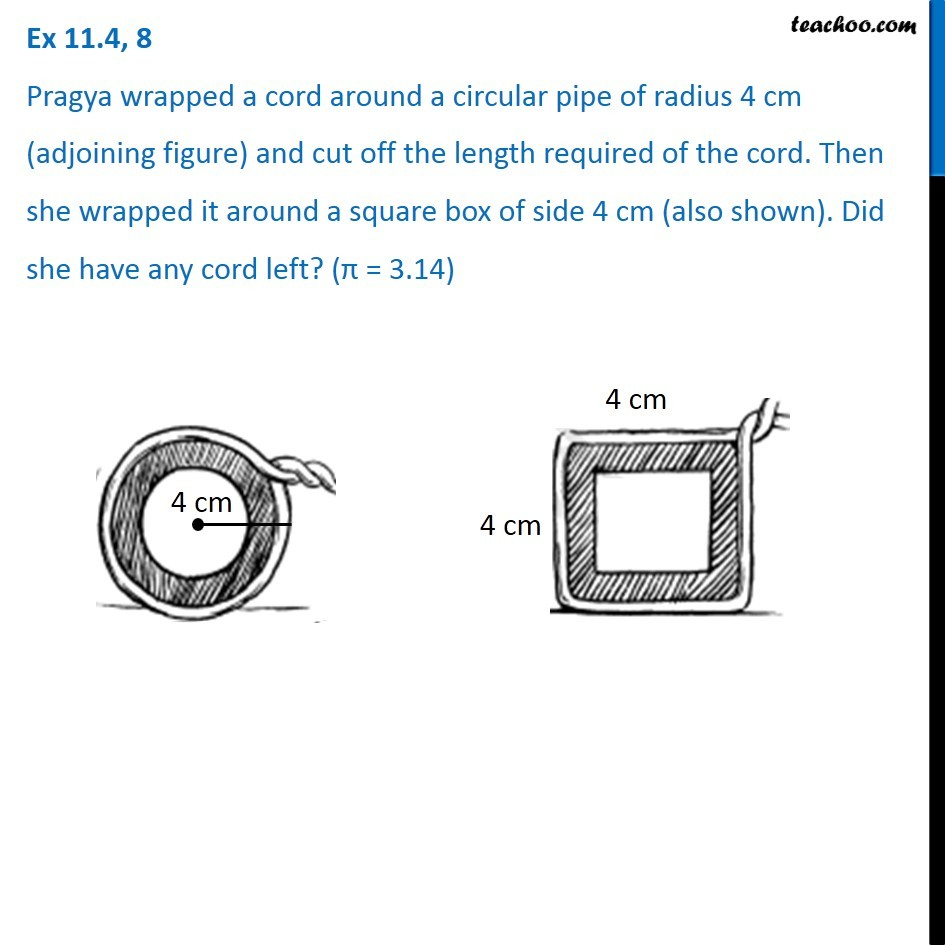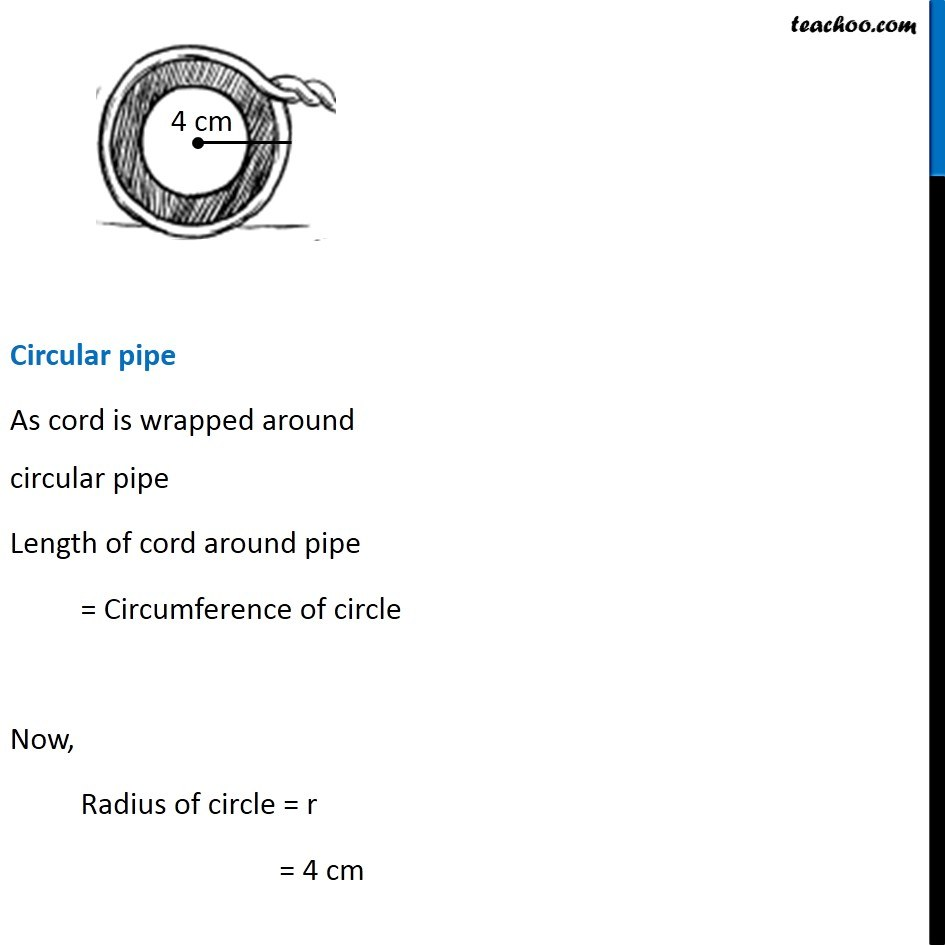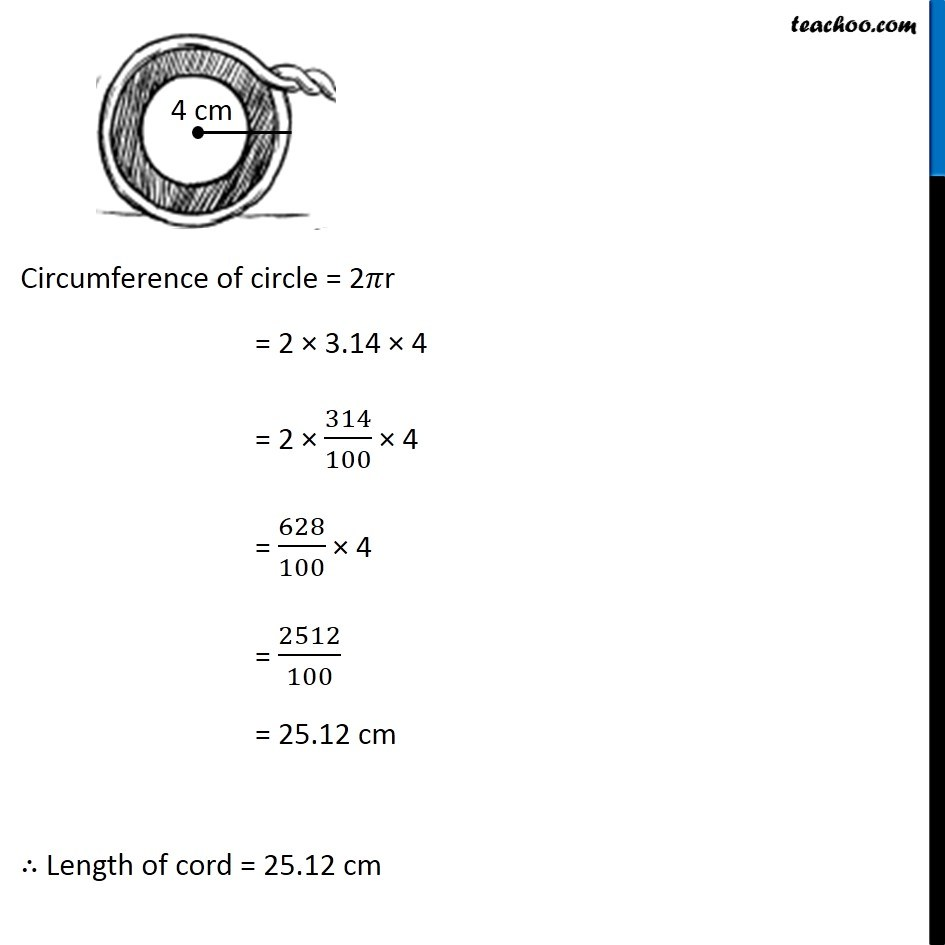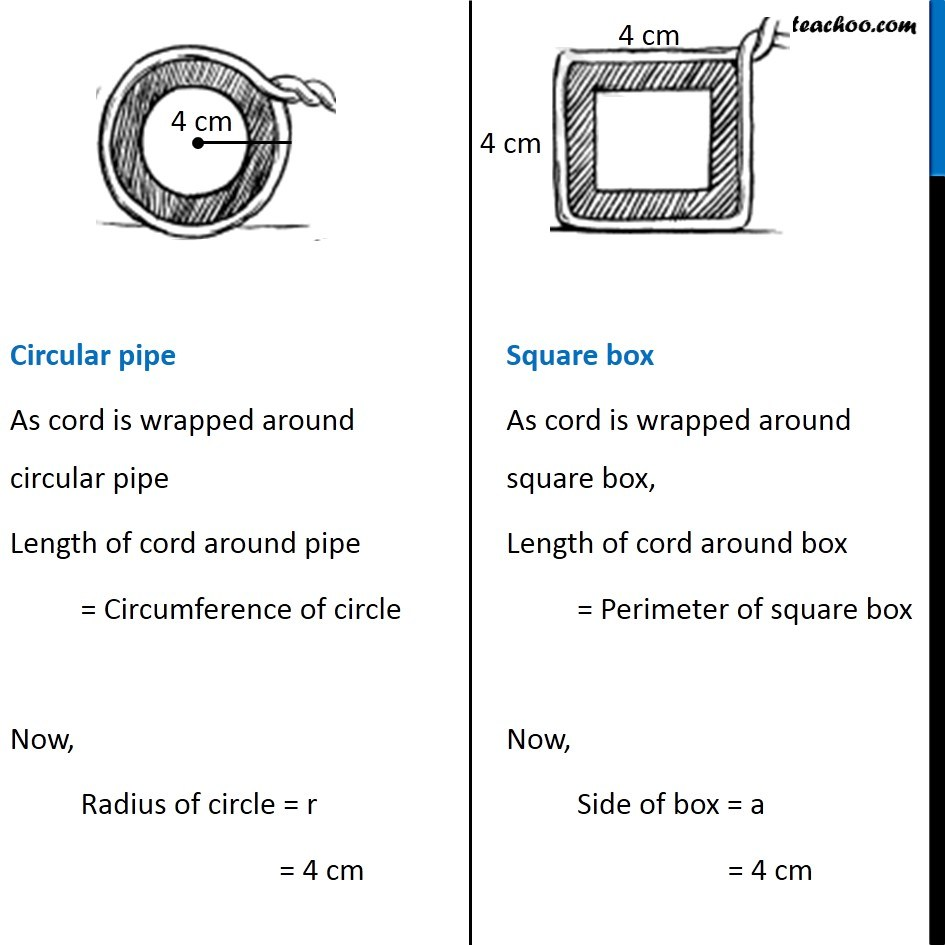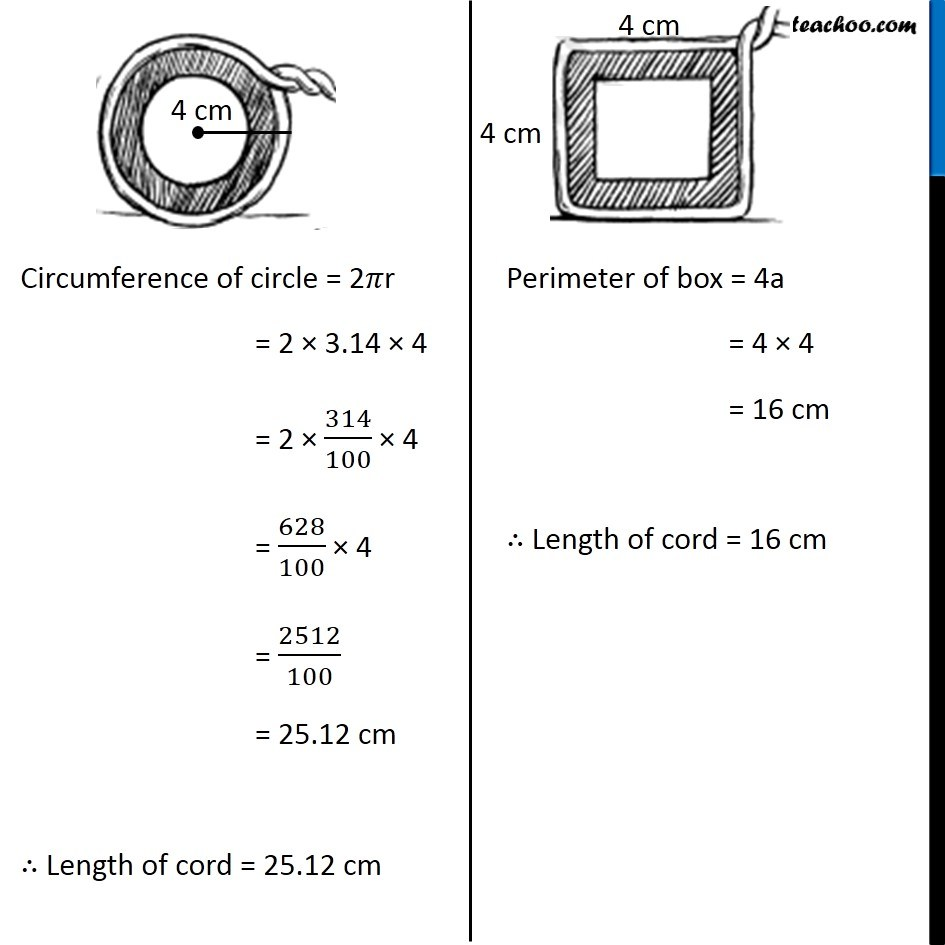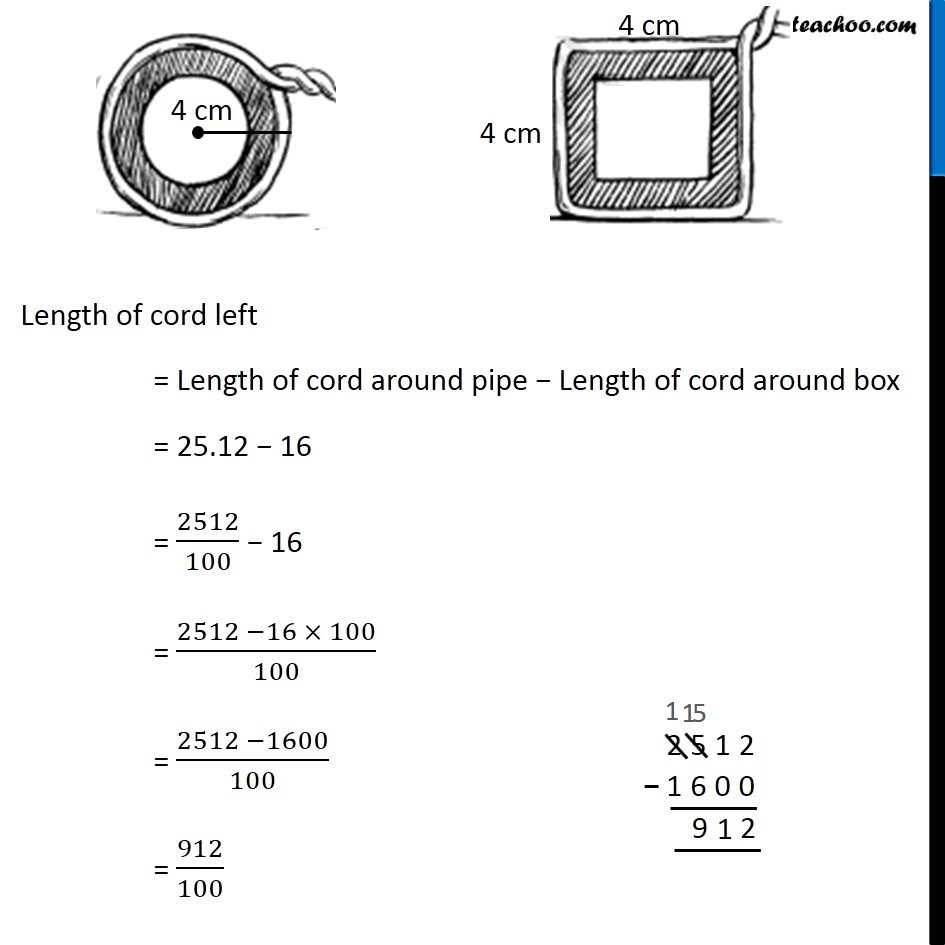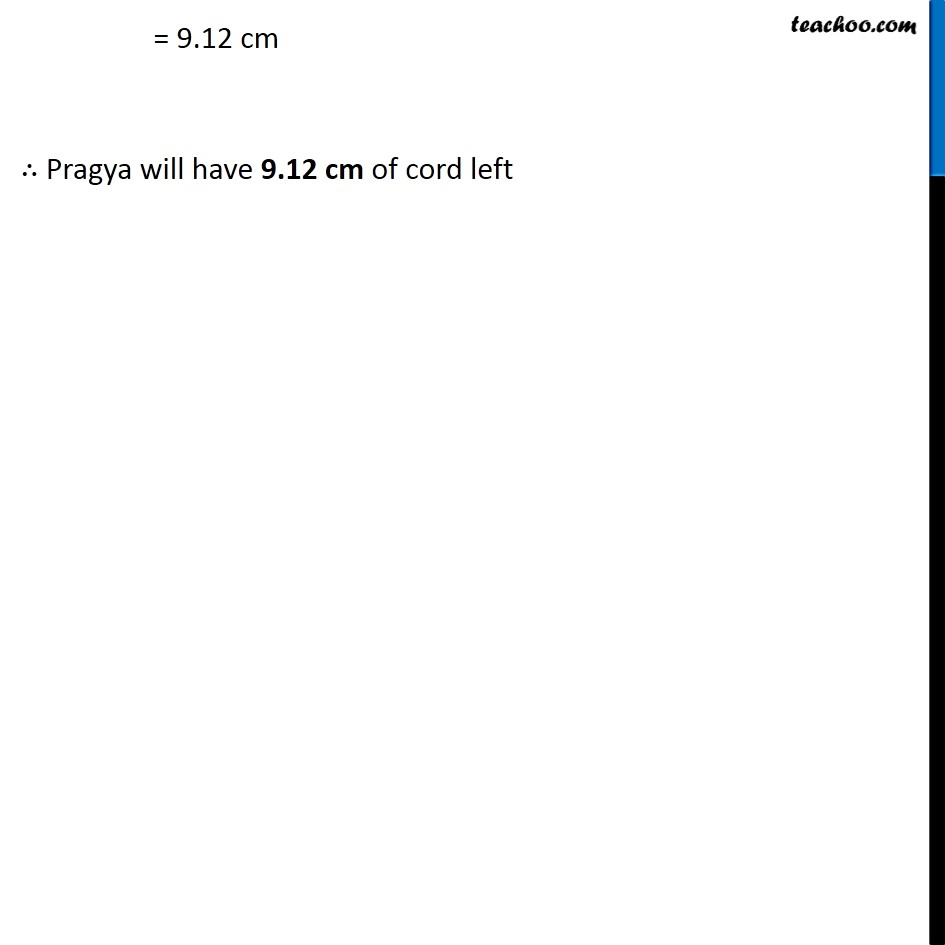Get live Maths 1-on-1 Classs - Class 6 to 12

### Transcript

Ex 11.4, 8 Pragya wrapped a cord around a circular pipe of radius 4 cm (adjoining figure) and cut off the length required of the cord. Then she wrapped it around a square box of side 4 cm (also shown). Did she have any cord left? (π = 3.14) Circular pipe As cord is wrapped around circular pipe Length of cord around pipe = Circumference of circle Now, Radius of circle = r = 4 cm Circumference of circle = 2𝜋r = 2 × 3.14 × 4 = 2 × 314/100 × 4 = 628/100 × 4 = 2512/100 = 25.12 cm ∴ Length of cord = 25.12 cm Circular pipe As cord is wrapped around circular pipe Length of cord around pipe = Circumference of circle Now, Radius of circle = r = 4 cm Square box As cord is wrapped around square box, Length of cord around box = Perimeter of square box Now, Side of box = a = 4 cm Circumference of circle = 2𝜋r = 2 × 3.14 × 4 = 2 × 314/100 × 4 = 628/100 × 4 = 2512/100 = 25.12 cm ∴ Length of cord = 25.12 cm Perimeter of box = 4a = 4 × 4 = 16 cm ∴ Length of cord = 16 cm Length of cord left = Length of cord around pipe − Length of cord around box = 25.12 − 16 = 2512/100 − 16 = (2512 −16 × 100)/100 = (2512 −1600)/100 = 912/100 = 9.12 cm ∴ Pragya will have 9.12 cm of cord left